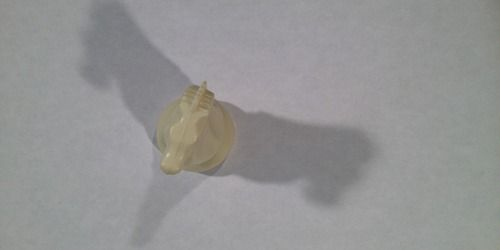## BlogResearchers have developed a new technique to measure the density matrix—a more general way of characterizing the state of a quantum system than that provided by the wave function.

The wave function is the physicist’s usual choice to characterize the state of a quantum system. But a different mathematical object, called a density matrix, is required for systems that are in mixed states, which are a mixture of other, pure quantum states. An example of a pure state is a beam of horizontally or vertically polarized photons, whereas a mixed state would be an uncorrelated statistical mixture of both polarizations. A mixed state would also apply to a system quantum mechanically entangled with its environment. The density matrix provides a complete description of a mixed state, but it also applies to pure states. Usually, experimental measurements of density matrices are indirect reconstructions using data acquired from a series of different kinds of measurements.